Ask your WordPress questions! Pay money and get answers fast!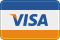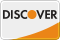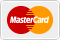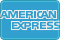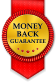# get_the_terms hierarchical WordPress

Okay, here's what I need:

I've been using get_the_term_list to output selected taxonomies like so:

```<?php echo get_the_term_list( get_the_ID(), 'howto', '<div class="category-nav four columns"><ul><li>','</li><li>','</li></ul>' ); ?> ```

This outputs a flat list of all terms selected. Works great.

But now I need that list to sort in a hierarchical fashion based on what's been selected.

Here's what I have now:

```function the_tax_list(\$taxterm,\$title,\$url){ \$myterm = \$taxterm; \$terms = get_the_terms(\$post->ID,\$myterm); if(\$terms){ ?> <div class="category-nav four columns"> <h2><?php echo \$title; ?></h2> <ul class="nobullet"> <?php foreach (\$terms as \$term) { if (0 == \$term->parent) { ?> <?php \$term_link = get_term_link( \$term, \$myterm ); ?> <li><a href="<?php echo \$term_link; ?>"><?php echo \$term->name; ?></a> <? \$subargs = array( 'orderby' => 'name', 'order' => 'ASC', 'hide_empty' => true, 'hierarchical' => true, 'child_of' => \$term->term_id, ); \$children = get_terms(\$myterm, \$subargs); ?> <? if( \$children ) { //if it has subcategories ?> <ul> <?php foreach(\$children as \$child){ ?> <?php \$term_link = get_term_link( \$child, \$myterm ); ?> <li><a href="<?php echo \$term_link; ?>"><?php echo \$child->name; ?></a></li> <?php } // end foreach?> </ul> <?php } // end if children?> <?php } // end if term has parent ?> </li> <? } // end foreach ?> <li class="all"><a href="<?php echo \$url; ?>">All</a></li> </ul> </div> <? } // end if terms } // end function```

Example usage:

`<?php the_tax_list('howto','How to','/libary/howto/'); ?>`

This outputs every <strong>child</strong> of a taxonomy, regardless of if it's selected for this particular \$post->ID.

I only want selected parents and their <em>selected</em> children.

2013-08-25

change this line

\$terms = get_the_terms(\$post->ID,\$myterm);

to

\$terms = get_the_terms(get_the_ID(),\$myterm);

in the fuction

so here is the full function again with required modification

```<?php function the_tax_list(\$taxterm,\$title,\$url){ \$myterm = \$taxterm; \$terms = get_the_terms(get_the_ID(),\$myterm); if(\$terms){ ?> <div class="category-nav four columns"> <h2><?php echo \$title; ?></h2> <ul class="nobullet"> <?php foreach (\$terms as \$term) { if (0 == \$term->parent) { ?> <?php \$term_link = get_term_link( \$term, \$myterm ); ?> <li><a href="<?php echo \$term_link; ?>"><?php echo \$term->name; ?></a> <? \$subargs = array( 'orderby' => 'name', 'order' => 'ASC', 'hide_empty' => true, 'hierarchical' => true, 'child_of' => \$term->term_id, ); \$children = get_terms(\$myterm, \$subargs); ?> <? if( \$children ) { //if it has subcategories ?> <ul> <?php foreach(\$children as \$child){ ?> <?php \$term_link = get_term_link( \$child, \$myterm ); ?> <li><a href="<?php echo \$term_link; ?>"><?php echo \$child->name; ?></a></li> <?php } // end foreach?> </ul> <?php } // end if children?> <?php } // end if term has parent ?> </li> <? } // end foreach ?> <li class="all"><a href="<?php echo \$url; ?>">All</a></li> </ul> </div> <? } // end if terms } // end function ?>```

I think this is mirroring what the code already did. This outputs <em>every</em> child of a taxonomy, regardless of if it's selected for this particular \$post->ID or using get_the_ID().

I only want <strong><em>selected</em></strong> parents and their <strong><em>selected</em></strong> children.

this function working fine , as it only provides you terms that are selected for post , have you tried it?

```function the_tax_list(\$taxterm,\$title,\$url){ \$myterm = \$taxterm; \$terms = get_the_terms(get_the_ID(),\$myterm); if(\$terms){ ?> <div class="category-nav four columns"> <h2><?php echo \$title; ?></h2> <ul class="nobullet"> <?php foreach (\$terms as \$term) { if (0 == \$term->parent) { ?> <?php \$term_link = get_term_link( \$term, \$myterm ); ?> <li><a href="<?php echo \$term_link; ?>"><?php echo \$term->name; ?></a> <?php \$subargs = array( 'orderby' => 'name', 'order' => 'ASC', 'hide_empty' => true, 'hierarchical' => true, 'child_of' => \$term->term_id, 'parent' => \$term->term_id ); \$children = get_terms(\$myterm, \$subargs); ?> <?php if( \$children ) { //if it has subcategories ?> <ul> <?php foreach(\$children as \$child){ ?> <?php \$term_link = get_term_link( \$child, \$myterm ); ?> <li><a href="<?php echo \$term_link; ?>"><?php echo \$child->name; ?></a></li> <?php } // end foreach?> </ul> <?php } // end if children?> <?php } // end if term has parent ?> </li> <?php } // end foreach ?> <li class="all"><a href="<?php echo \$url; ?>">All</a></li> </ul> </div> <?php } // end if terms } // end function ?>```

2013-08-26